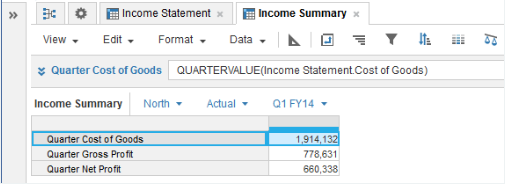1. Calculation functions
2. All Functions
3. Time and Date Functions
4. QUARTERVALUEReturns the quarter value of the source line item or property that matches the time Summary Method set for the source.

## Syntax

`QUARTERVALUE(x) `

where:

• x: Line item or property

## Format

Input Format Output Format

xLine item - number, Boolean, date, time period, list, text.

Matches the input format.

## Arguments

The function uses the following arguments:

• x: Number, Boolean, date, time period, list item, text.

## Constraints

The function has the following constraints:

• The result formatting must match the source formatting.
• The result must have a timescale applied.
• Quarter Totals must be selected in the model calendar.
• The time period granularity of the source must be quarter, month, week, or day. If you make the source time period granularity half-year, for example, QUARTERVALUE returns zero.
• If the time summary method is none, only numbers get values. Use other time summary methods to get values for Booleans, dates, time periods, list items, and text. See Summary Methods and Time Aggregation for more information.

## Excel equivalent

• No Excel equivalent

## Examples

#### Sum example

The Product R&D Score number-formatted source line item has Sum selected for the Time Summary method: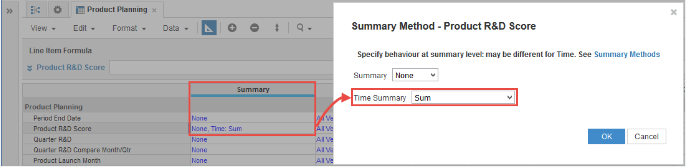QUARTERVALUE returns the summed values for each quarter: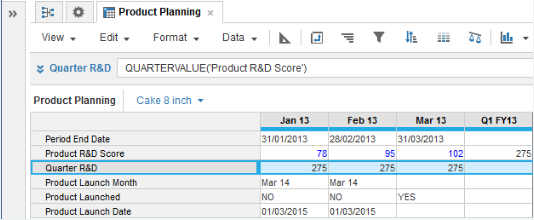#### Average example

If the Product R&D Score number-formatted source line item has Average selected for the time summary method, you can use QUARTERVALUE to return the average value for each quarter.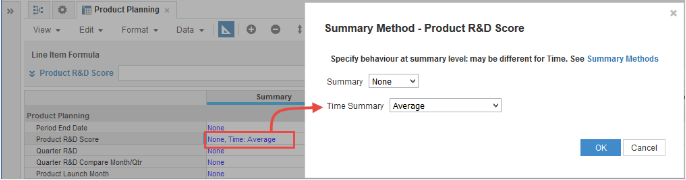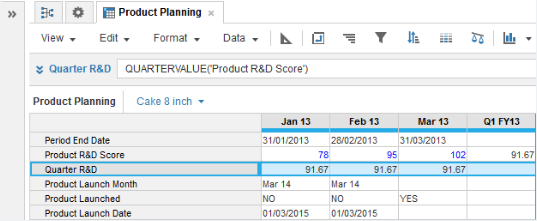#### Min example

If the Product R&D Score number-formatted source line item has Min selected for the time summary method, you can use QUARTERVALUE to return the minimum value for each quarter: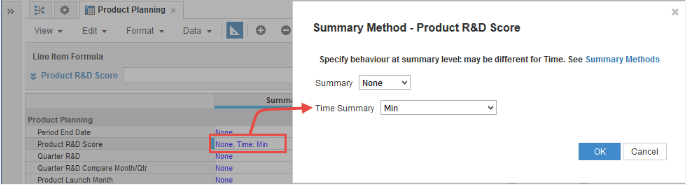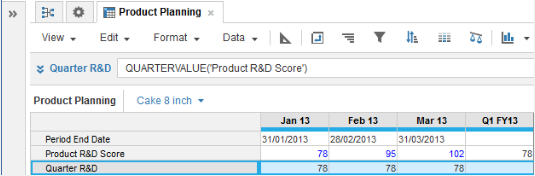#### Example

If the Product R&D Score number-formatted source line item has Sum selected for the time summary method and the time period granularity of the source is days the Product R&D Score is summed for the quarter.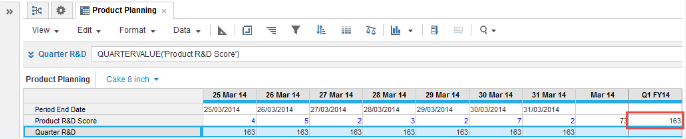#### Example

In this example, an Income Statement source module shows gross and net profit by region for Actual and Forecast versions: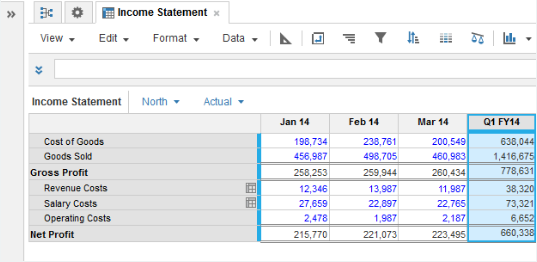The time summary method for the Cost of Goods, Gross Profit, and Net Profit line items is set to Sum: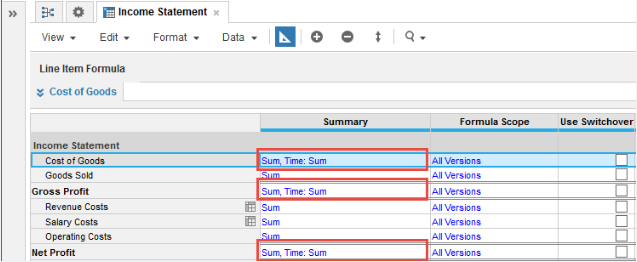In an Income Summary results module with a timescale applied, QUARTERVALUE is used in number-formatted results line items to pull-in any required quarter totals: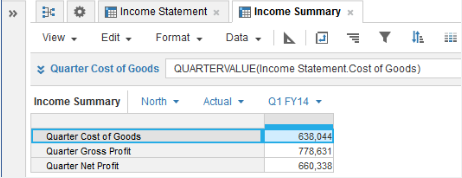Ensure that the time summary method you select for the results line items gives you the values that you want.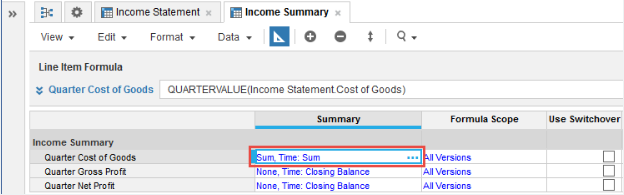In this example, If you change the time summary method for Quarter Cost of Goods from Closing Balance to Sum, the value returned for Q1 FY14, where the result line item timescale is set to Months, is the multiple of the each month's quarter values:

3 x 638,044 = 1,914,132: